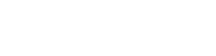October 7, 2023
October 7, 2023
October 7, 2023
###### Environment
October 7, 2023
 Question 4

Let G = (V,E) be a directed graph with n vertices. A path from vi to vj in G is sequence of vertices (vi, vi+1, ……., vj) such that (vk, vk+1) ∈ E for all k in i through j-1. A simple path is a path in which no vertex appears more than once. Let A be an nxn array initialized as follow.Consider the following algorithm.

```for i = 1 to n
for j = 1 to n
for k = 1 to n
A [j,k] = max (A[j,k] (A[j,i] + A [i,k]);```

Which of the following statements is necessarily true for all j and k after terminal of the above algorithm?

 A A[j,k] ≤ n B If A[j,j] ≥ n-1, then G has a Hamiltonian cycle C If there exists a path from j to k, A[j,k] contains the longest path length from j to k D If there exists a path from j to k, every simple path from j to k contains at most A[j,k] edges
Question 4 Explanation:Here, we have two functionalities i.e., from j to k, there exists a path then it results otherwise 0.
If there exist a path then it results
A[j,k] = max(A[j,k], A[j,i]+A[i,k])
i.e., if there exists a path from j to k, every simple path from j to k contains the atmost A[j,k] edges.
Question 4 Explanation:Here, we have two functionalities i.e., from j to k, there exists a path then it results otherwise 0.
If there exist a path then it results
A[j,k] = max(A[j,k], A[j,i]+A[i,k])
i.e., if there exists a path from j to k, every simple path from j to k contains the atmost A[j,k] edges.